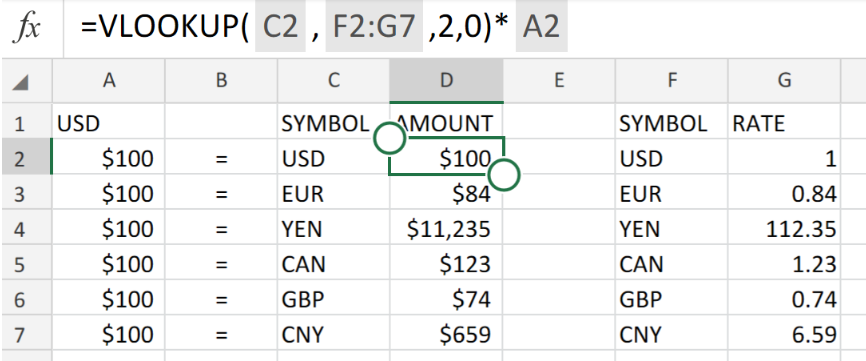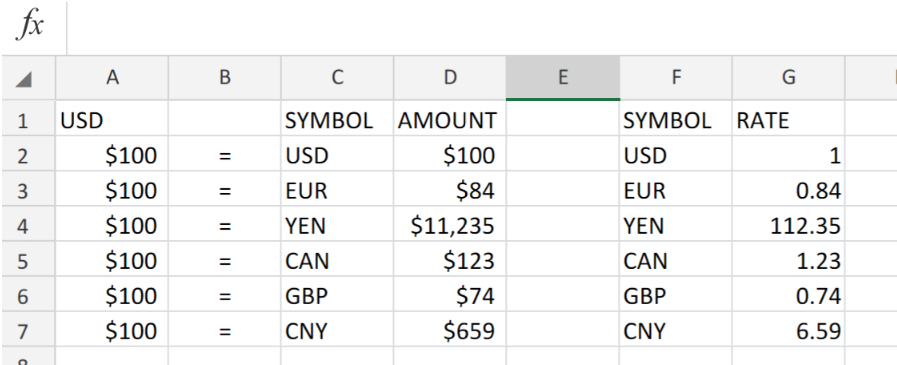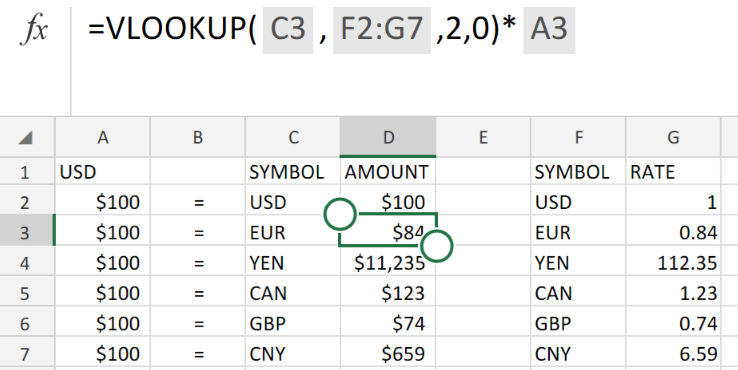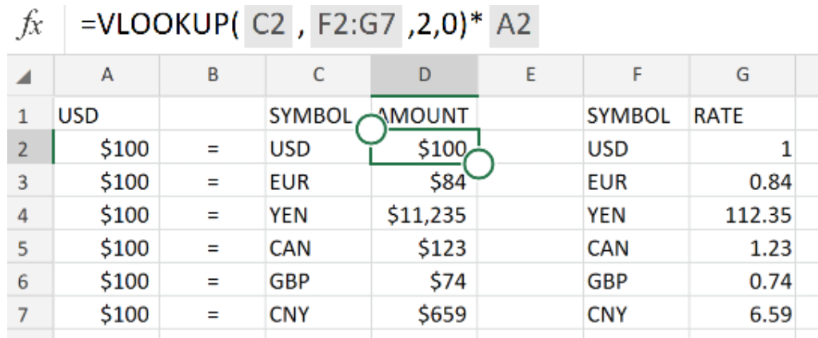Get instant live expert help with Excel or Google Sheets“My Excelchat expert helped me in less than 20 minutes, saving me what would have been 5 hours of work!”

#### Post your problem and you’ll get expert help in seconds.

Your message must be at least 40 characters
Our professional experts are available now. Your privacy is guaranteed.

# Simple currency conversionFigure 1. of Currency Conversion in Excel.

In order to convert a Currency to other specified currencies, we can utilize the Excel VLOOKUP Function.

The Excel VLOOKUP Function operates by using various country Currency Codes that we are required to provide.

## Generic Formula.

`=VLOOKUP(currency,xtable,column,0)*amount`

Note – xtable is the range of cells for a second table which specifies the current Currency Exchange Rates.

## How to use the Excel Currency Conversion Function.

We can utilize The Excel VLOOKUP Function to help us in converting USD amounts to other currencies by using their currency codes.

We can carry out this operation in the following simple steps!

1. Set up your Excel worksheet with the relevant details.

Provide separate columns for the currency symbol and current Currency Conversion Rates. This will be our xtable. See figure below;Figure 2. of Simple Currency Conversion in Excel.

1. Click on cell D2 in the AMOUNT column and enter the operation syntax provided below in the formula bar of our worksheet.

`=VLOOKUP(C2,F2:G7,2,0)*A2`

This will convert the amounts located in the USD column into the currency we have indicated the SYMBOL column.

Note – our xtable cell range for this illustration is F2:G7

1. Copy the operation syntax into the next cell D3 of the AMOUNT column as displayed below;Figure 3. of Simple Currency Conversion in Excel.

The Vlookup operation syntax locates the currency in the Excel Table, and then retrieves the current Currency Conversion Rate from column G and converts the USD amount.

The basis of this operation is the VLOOKUP Function.Figure 4. of Final Result.

## Instant Connection to an Expert through our Excelchat Service:

Our live Excelchat Service is here for you. We have Excel Experts available 24/7 to answer any Excel questions you may have. Guaranteed connection within 30 seconds and a customized solution for you within 20 minutes

### Did this post not answer your question? Get a solution from connecting with the expert.Another blog reader asked this question today on Excelchat:
Solution examplesI have a pivot table in which per order (on the rows) stands how much products they ordered per size (on the columns). I want to determine which combinations of quantities of sizes people order. And I want to count these combinations.
Solved by E. W. in 60 minsI have 500 numbers in column A with aproximatly 15digits. In column B I have 5 numbers with 6 digits Can a find a formula wich can transfer all numbers from A column wich start with some 6 digitsa from B column
Solved by V. L. in 32 minsI have a sheet with 3 columns. First column is a code for records in column B (has 563 records). What I need is a formula to show me if what is in column C (has 4400 records) is in column B and if it is to take the code for that record. Example: Column A(code corresponding to column B): 12, 14, 15, 19 Column B(names): asd, adf, ade, aqw Column C(names): akd, adf, ade, anb, wgs I need something that would say, if record in column C (for example adf) is matching record in column B (adf) return the code from column A (the code corresponding to that record in column B),if not return blank.
Solved by C. J. in 8 minsIf the data in A matches the data in C, then I need the data in E to be placed in B
Solved by X. W. in 40 minsI have a workbook with 2 sheets. First sheet has column A which is a device name, then columns B - infinity that have numeric "tags" for that device. Each device can have one or 20 "tags", tags are all numerical. Worksheet 2 has column A which is numeric value and B which is a human readable value of what the tag is. Question is, how do I replace all the tag numbers in worksheet 1 with the actual human readable values is sheet 2?
Solved by T. H. in 16 mins## Subscribe to Excelchat.coAnother blog reader asked this question today on Excelchat: